Topics: Implementation Support

# Read-Draw-Write: Making Word Problems Less Problematic

February 10, 2021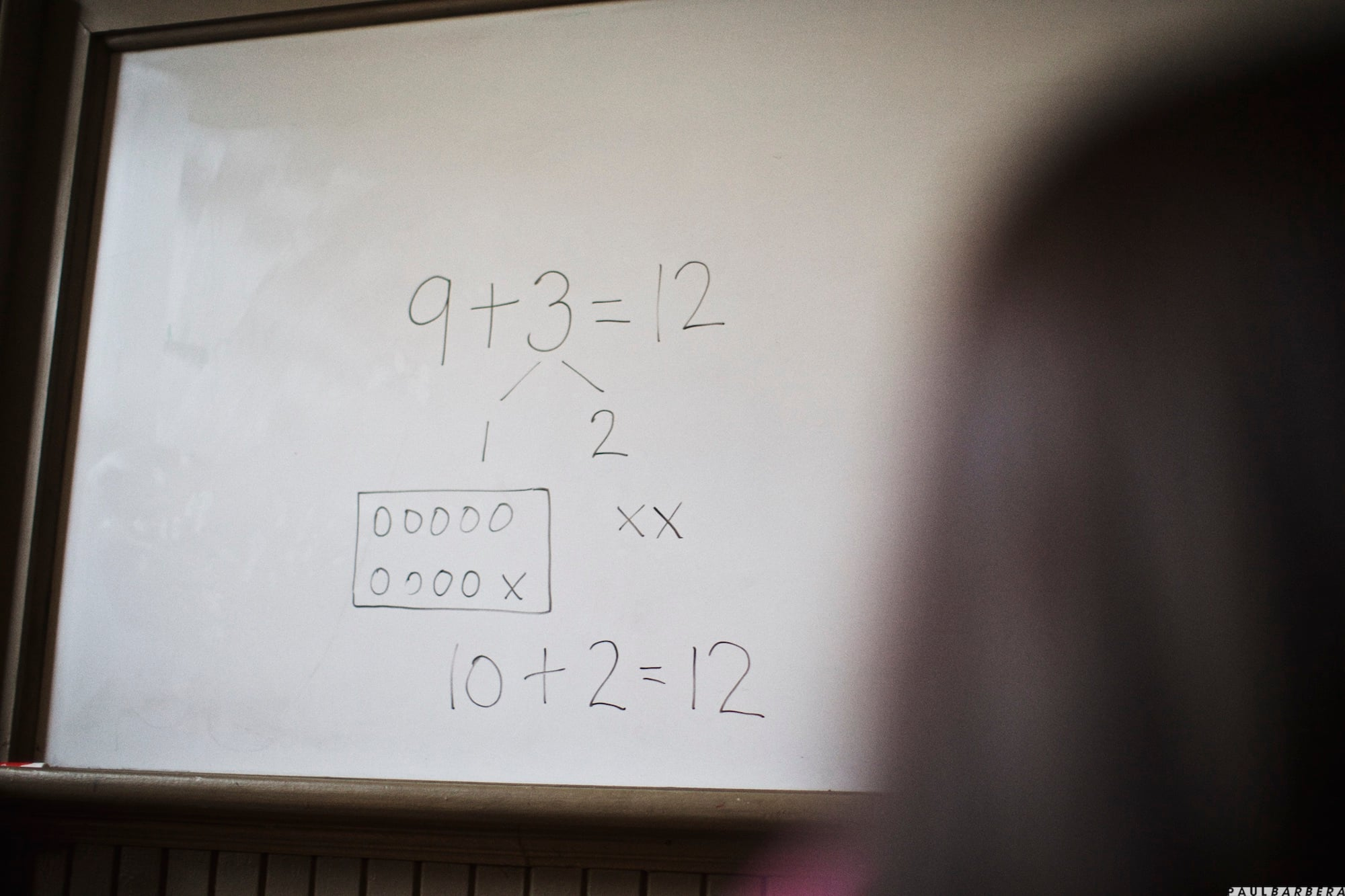Posted in: Aha! Blog > Eureka Math Blog > Implementation Support > Read-Draw-Write: Making Word Problems Less Problematic

Students need a better approach to word problems than just key words.

If you’re anything like me, teaching students to solve word problems can be challenging at times. You pose a word problem only to find from your students’ work that they have misinterpreted what’s actually happening in the problem. Consider the following Grade 1 problem:

Nine dogs were playing at the park. Some more dogs ran in. Then there were 12 dogs in all. How many dogs ran in?

Student Response:

9 + 12 = 21

Twenty-one dogs ran in.

The student likely looked through the problem and saw the numbers 9 and 12. Since they saw the word more, they decided to add. This is a common mistake that comes from using a key word approach to word problems. Thankfully, there’s a more effective approach. Eureka Math® encourages students to use the Read–Draw–Write (RDW) process as a tool for solving word problems.

The RDW process helps students make sense of word problems.

Students using the RDW process first read the whole problem to get an idea of what’s happening in the story. Then, they alternate between reading and drawing until the drawing helps them understand the problem and see how to solve it. Finally, students solve the problem and write the answer. The example shows a tape diagram, but students can use other types of drawings such as number bonds or circles to represent items in a problem.

 Read Draw and Write Nine dogs were playing at the park.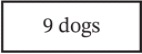Some more dogs ran in.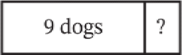Then there were 12 dogs in all.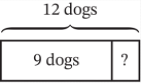How many dogs ran in? 9 + ? = 12 ? = 3 Three dogs ran in.

Encourage students to draw each part as they read it. Emphasize to students that drawing helps them make sense of both the situation and the math in a word problem. Drawings also serve as a way for students to show their work and are usually accepted as explanations, even on high-stakes tests.

Students can use the RDW process as a solution strategy across grade levels.

Students can use the RDW process in all grade levels. The strategy works when solving math problems from elementary school through high school. For example, consider this SAT®-style question.

A clothing design business makes 3 million more dresses the second year than the first. The third year, the business makes double the number of dresses it made the second year. If the business makes 38 million dresses the third year, how many dresses, in millions, did it make the first year?

Students might be unsure how to make sense of the problem or even know where to begin. By applying the RDW process, students can read the problem, represents chunks of the problem with drawings, and get to a solution.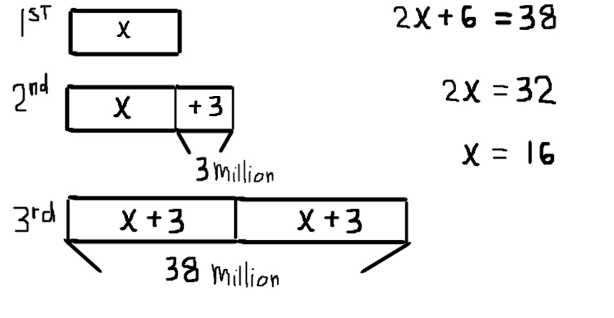The drawings help students get past the complexity of the problem. Students can then see how to represent the problem by using an algebraic equation they can solve.

Cultivate mathematical thinking by using the RDW process.

To help students become familiar with the RDW process, start by modeling the steps. Read the whole problem aloud. Then chunk the problem. Ask, “Can I draw something? What can I draw?” Model different types of drawings based on student responses (even for the same problem) to show students there is more than one correct way to represent a problem. Call out how you keep going back to reread the problem, how you add to a drawing, or how you change a drawing when you think a different type of drawing is more helpful.

After modeling the RDW process for students, move on to guided practice. Though you guide the process, students work independently at times and then come back together.

• Have students read the problem. Then ask what they think the most important chunks of information are to represent in a drawing.
• Give students time to draw. Then have them work with a partner to critique one another’s work.
• Have students solve the problem independently. Then have them work with their partner to check their solutions.
• Debrief by selecting different students’ work to share and discuss as a class.

Once you guide students through the RDW process, they can engage in independent practice. While students work independently, move around the room and ask questions of individual students to help them think about their work. Consider which student solutions to share and discuss as a class. Reinforce the value of using the RDW process by asking questions about how students represented the problem and how drawings helped lead to a solution. Call out how students represented the same information differently. Ask students what they see in someone else’s work that they would consider doing next time. When you cultivate this culture of mathematical discourse, students feel safe exploring different strategies for problem solving.

The RDW process creates a bridge from solution process to conceptual understanding. This strategy stays with students through their school years and empowers them to tackle new math problems with confidence.

Topics: Implementation Support